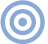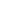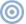# Reference

## The Jitter Game The Jitter Game Revisited, April 1993

The Jitter Game Revisited, April 1993 (Vol.16 No.4):

This "Follow-Up" also provides an opportunity to clarify a perplexing issue raised in the January jitter article. From those measurements, it would appear that processors with single-bit converters have much lower jitter than the more common 8x-oversampling units that use multi-bit DACs. Indeed, 1-bit converters typically have only a few picoseconds of jitter, rather than several hundreds of picoseconds or even several nanoseconds for multi-bit converters. Is the jitter performance of 1-bit machines intrinsically lower, thus "better"?

Yes and no. On an absolute scale, the 1-bit DACs did indeed have vastly lower measured jitter. But the single jitter figure in isolation doesn't tell the whole story. The jitter level must be considered in relation to the clock frequency: 1-bit converters have very fast word clocks which run at some high multiple of 44.1kHz. These clock frequencies are typically 128x (128 times 44,100, or 5.645MHz), 192x (8.467MHz), 256x (11.289MHz), or 384x (16.934MHz). Multi-bit converters have a much slower word clock, generally running at 8x the sampling rate, or 352.8kHz.

Now, it is intuitive that 100ps of jitter on a 16.9MHz clock is a far greater error than 100ps on a 352.8kHz clock. The 16.9MHz clock has a period (the time it takes to complete one cycle) of only 59ns; the 352.8kHz 8x clock has a period of a leisurely 2800ns or 2.8µs (48 times slower). A timing variation of 100ps is huge in relation to the 59ns period, but very small compared to the relatively long 2.8µs period of the 352.8kHz clock. Put another way, the 100ps is a much larger percentage of the period for the faster clock, and thus more significant (footnote 1).

It therefore seems appropriate to express jitter in relation to the clock frequency. Rather than ask readers to calculate the clock period from the oversampling rate and figure out the relative jitter levels for themselves, we have decided to normalize all jitter measurements to the very common 8x-oversampling clock found in virtually all current multi-bit CD players and D/A converters.

Here's how it works. Say we measure 3ps of jitter on a 384x clock. Rather than ask you to extrapolate the figure to an 8x-oversampling converter for comparison, we will perform the conversion. First, we find the ratio between 8x-oversampling and 384x (48), and multiply the measured 3ps by that ratio. This gives us an answer of 144ps, or the 8x-oversampling equivalent of 3ps on a 16.9MHz clock. Note that the ratio between the jitter deviation and the clock frequency is identical for 3ps of jitter on a 384x clock and 144ps on an 8x-oversampling clock.

Normalizing all jitter measurements to an 8x clock makes it possible to directly compare jitter measurements on products with a variety of clock frequencies.

The Sumo Theorem provides an excellent example of how normalizing the jitter measurements to an 8x-oversampling clock works. The Theorem's PCM67 DAC is a hybrid, with a multi-bit DAC handling the upper ten bits and a 1-bit DAC for the lower eight bits. The chip is fed both a high-speed clock (384x-oversampling, or 16.934MHz) and an 8x-oversampling clock (352.8kHz). Measuring the jitter at the high-speed clock revealed 5.89ps of RMS jitter with a full-scale input signal. But at the 8x clock, the measured jitter was 371ps. Does the Theorem have extraordinarily low jitter of less than 6ps, or is it more in line with the average jitter performance of 371ps? By multiplying 5.89ps by 48 (the ratio between a 384x clock and an 8x clock), we get 283ps, slightly lower than the 371ps measured at the 8x clock (the higher jitter at the 8x clock is probably a result of dividing the master 16.9MHz clock to obtain the 352.8kHz clock). Just as the clock frequency is divided down, so, too, is the jitter (footnote 2).

Again, note that 5.89ps is to 16.9MHz what 282ps is to 352.8kHz; the ratio between the jitter and the clock period is identical. In all future jitter measurements of processors with oversampling rates higher than 8x, the jitter figures presented will include the words "normalized to an 8x-clock frequency."

If you didn't follow all of that, don't worry. Just remember that when you see a jitter measurement presented, you don't need to calculate the jitter in relation to the clock frequency—it has been factored in to the measurement result.—Robert Harley

Footnote 1: It has been suggested in discussions I've had with designers that jitter is a much more sonically significant factor in 1-bit than in multi-bit converters.—Robert Harley

Footnote 2: We chose the higher jitter figure measured at the 8x clock because this is the clock that actually controls the conversion of digital to analog.—Robert HarleyARTICLE CONTENTSX